• 2．学会利用MATLAB函数对离散时间信号和系统的频域进行计算。 二、实验原理： 1.离散时间傅里叶变换（DTFT） 序列的离散时间傅里叶变换（DTFT）定义为： 通常是实变量Ω的复函数。实例程序演示如下： 【例3.1】求...

一、实验目的：

1．掌握离散时间信号和系统的频域分析方法；
2．学会利用MATLAB函数对离散时间信号和系统的频域进行计算。

二、实验原理：

1.离散时间傅里叶变换（DTFT）

序列的离散时间傅里叶变换（DTFT）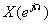定义为：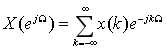通常是实变量Ω的复函数。实例程序演示如下：
【例3.1】求有限长序列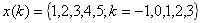的DTFT，并画出它的幅度谱，相位谱，实部和虚部。

clear all
x=[1,2,3,4,5];
k=-1:3;
w=linspace(0,2*pi,512);
H=x*exp(-j*k'*w);
subplot(2,2,1);plot(w,abs(H));ylabel('幅度');     %画幅度特征曲线
subplot(2,2,2);plot(w,angle(H));ylabel('相角');   %画相位特征曲线
subplot(2,2,3);plot(w,real(H));ylabel('实部');    %画幅度实部特征曲线
subplot(2,2,4);plot(w,imag(H));ylabel('虚部');   %画幅度虚部特征曲线

其程序运行结果如下：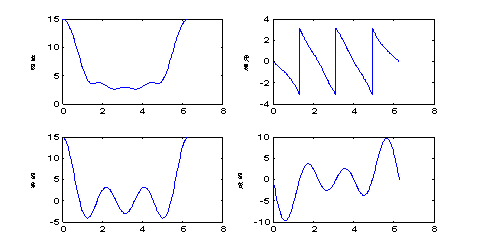2.离散LTI系统的频率响应

利用MATLAB提供的freqz函数可以计算离散信号的频谱或离散系统频率响应的抽样值。若信号的频谱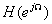表示为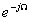的有理多项式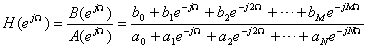则freqz函数其调用格式为：

X=freqz(b,a,w)

其中，X为系统频谱，w是抽样的频率点，b和a分别为离散LTI系统的系统函数的H(z)的分子和分母多项式系数。
说明：不带输入向量时freqz函数将自动绘出频率响应的幅频和相频特性曲线。
【例3.2】已知离散系统的H（z）为：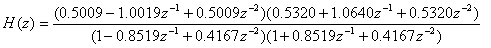试画出该系统的幅度响应。

程序如下：
clear all
b1=[0.5009 -1.0019 0.5009];
b2=[0.5320 1.0640 0.5320];
a1=[1 -0.8519 0.4167];
a2=[1 0.8519 0.4167];
b=conv(b1,b2);  %计算H（z）的分子多项式系数
a=conv(a1,a2);  %计算H（z）的分母多项式系数
w=linspace(0,pi,512);
h=freqz(b,a,w);
plot(w/pi,abs(h));

运行结果：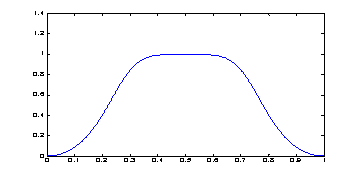3.对X(z)部分分式展开

MATLAB提供了计算序列z变换X(z)的部分分式展开函数，其调用格式为：
[r,p,k]=residuez(b,a)
其中， b和a分别为用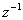表示X(z)的分子和分母多项式的系数。 若 X(z)的部分分式展开为：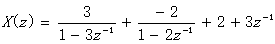则residuez的返回参数r,p,k分别为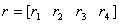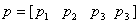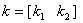residuez也可以用于由 r,p,k计算表示X(z)的分子和分母多项式的系数，其调用格式为：

[b,a]=residuez(r,p,k)

1. X(z)的零极点函数
函数zplane可以画出X（z）的零极点坐标图，其调用格式是：

zplane(b,a)

[例3.3]试用MATLAB计算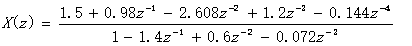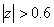的部分分式展开。并画出X（z）的零极点图。
程序如下：

clear all
b=[1.5,0.98,-2.608,1.2,-0.144];
a=[1,-1.4,0.6,-0.072];
[r,p,k]=residuez(b,a);
disp('留数');disp(r');
disp('极点');disp(p');
disp('常数');disp(k);
zplane(b,a);

程序的运行结果为：
留数
0.7000 0.5000 0.3000
极点
0.6000 0.6000 0.2000
常数
02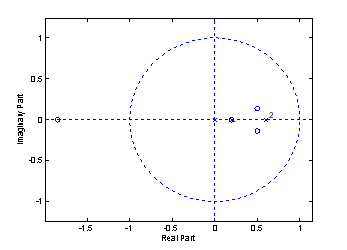三、作业：

1.已知序列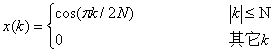画出该序列DTFT的实部、虚部、幅度谱和相位谱。并分析的特点。

clear all
k=-10:10;
x=cos(pi*k/20);
w=linspace(0,2*pi,512);
H=x*exp(-j*k'*w);
subplot(2,2,1);plot(w,abs(H));ylabel('幅度');     %画幅度特征曲线
subplot(2,2,2);plot(w,angle(H));ylabel('相角');   %画相位特征曲线
subplot(2,2,3);plot(w,real(H));ylabel('实部');    %画幅度实部特征曲线
subplot(2,2,4);plot(w,imag(H));ylabel('虚部');   %画幅度虚部特征曲线

2.利用MATLAB函数计算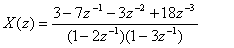的部分分式展开的各子系统的分子、分母多项式的系数。根据计算结果写出表达式。

clear all
b=[3,-7,-3,18];
a1=[1,-2];
a2=[1,-3];
a=conv(a1,a2);
[r,p,k]=residuez(b,a);
disp('留数');disp(r');
disp('极点');disp(p');
disp('常数');disp(k);
zplane(b,a);

留数
3.0000 -2.0000

极点
3.0000 2.0000

常数
2 33.离散稳定的LTI系统的差分方程为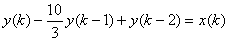，画出该系统的频率响应函数，幅度谱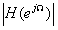，相位谱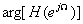及其零极点图。

clear all
b=;
a=[1,-10/3,1];
w=linspace(-pi,pi,512);
h=freqz(b,a,w);
subplot(1,2,1);plot(w/pi,abs(h));ylabel('幅度');     %画幅度特征曲线
subplot(1,2,2);plot(w/pi,angle(h));ylabel('相角');   %画相位特征曲线

clear all
b=;
a=[1,-10/3,1];
[r,p,k]=residuez(b,a);
disp('留数');disp(r');
disp('极点');disp(p');
disp('常数');disp(k);
zplane(b,a);

更多相关文章点这里哦

数字信号处理----全套Matlab实验报告

通信工程(信息类，电子类，电气工程，自动化，计算机，软件工程，机电，等相关专业)全套学习指导

展开全文matlab 数字信号处理 离散时间信号 频域分析
• 强大的 MatLAB 函数，通过将其傅立叶频谱与 -iw（w = 循环频率）相除，在频域中对离散时间信号进行积分； 另请参阅 diffFD（频域微分函数）。matlab
• 基于MATLAB的语音信号频域特征分析，给出设计原理、过程以及MATLAB程序。
• 语音信号频域分析包括语音信号的频谱、功率谱、倒频谱、频谱包络分析等,而常用的频域分析方法有带通滤波器组法、傅里叶变换法、线性参见测法等几种。本文采用傅里叶变换法结合matlab讨论了一段语音信号的短时谱、...

语音信号的频域分析包括语音信号的频谱、功率谱、倒频谱、频谱包络分析等,而常用的频域分析方法有带通滤波器组法、傅里叶变换法、线性参见测法等几种。本文采用傅里叶变换法结合matlab讨论了一段语音信号的短时谱、语谱图、倒谱和复制谱等特征,给出了基音周期及共振峰等仿真结果。

2 1年 8月 01

湖北第二师范学院学报

J un lo b iUmv mi o u a o o r a fHu e e￣ fEd c t n i

Al 2 1 唱. 0 1

第 2卷第 8 8期

V0. 8 No 8 12 .

基于 MA L B分析语音信号频域特征 TA

肖正安

(北第二师范学院物理与电子信息学院，汉 4 00 )湖武 3 25

摘要：语音信号的频域分析包括语音信号的频谱、功率谱、倒频谱、频谱包络分析等，常用的频域分析方法有带通滤而

波器组法、里叶变换法、傅线性参见测法等几种。本文采用傅里叶变换法结合 m tb讨论了一段语音信号的短时谱、 aa . 1语

谱图、倒谱和复制谱等特征，出了基音周期及共振峰等仿真结果。给

关键词： T A频谱分析；时傅里叶变换； MA L B;短复倒谱中图分类号：N 1. T 92 3文献标识码： A文章编号：6 434 2 1 ) 80 3 -3 17 -4 X(0 1 0 -( 50 2

作者简介：肖正安( 96一)男， 17,湖北钟祥人，讲师，硕士，究方向为数字信号处理及通信电子线路。研

1引言

假定

信号的傅立叶表示在信号的分析与处理中起着重要的作用。因为对于线性系统来说，可以很方便地确

定其对正弦或复指数和的响应，以傅立叶分析方法所

( ) =.

()( je J —},} )’础

(.) 24

则可以得到

能完善地解决许多信号分析和处理问题。另外，傅立叶表示使信号的某些特性变得更明显，因此，它能更深入地说明信号的各项物理现象。 由于语音信号是随着时间变化的，通常认为，语音

瓦 ( ) e嚣( ) = (.) 25同样，不同的窗口函数，将得到不同的傅立叶变换

式的结果。由上式可见，短时傅立叶变换有两个变量： n∞所以它既是时序 1的离散函数，和，又是角频率∞

的连续函数。与离散傅立叶变换逼近傅立叶变换一是一个受准周期脉冲或随机躁声源激励的线性系统的样，如令∞=,/则得离散的短时傅立叶吧如下： 2r N, r k 输出。输出频谱是声道系统频率响应与激励源频谱的 x( n )= n k X ( )=乘积。声道系统的频率响应及激励源都是随时间变化的，因此一般标准的傅立叶表示虽然适用于周期及平

( 1 1—m)一 m)1 ' 3t ( e

J= n

一∞

,0≤Ⅳ一1 (≤ ) ( .) 26

稳随机信号的表示，但不能直接用于语音信号。由于

语音信号可以认为在短时间内，近似不变，因而可以采 22语谱图 .用短时分析法。 水平方向是时间轴，垂直方向是频率轴，图上的灰本文从傅里叶分析原理出发，编写程序估计短时度条纹代表各个时刻的语音短时谱。语谱图反映了语

谱、倒谱，画出语谱图，并分析仿真结果，在此基础上， 借助频域分析方法所求得的参数分析语音信号的基音

周期或共振峰。 2语音分析基本方法

2 1短时傅立叶变换 .

音信号的动态频率特性，在语音分析中具有重要的实用价值。被成为可视语言。 语谱图的时间分辨率和频率分辨率是由窗函数的特性决定的。时间分辨率高，以看出时间波形的每可个周期及共振峰随时间的变化，但频率分辨率低，不足

由于语音信号是短时平稳的随机信号，某一语音信号帧的短时傅立叶变换的定义为：

( ) ∑ ( (— e = m) n m)

(. ) 2 1

以分辨由于激励所形成的细微结构，称为宽带语谱图； 而窄带语谱图正好与之相反。 宽带语谱图可以获得较高的时间分辨率，反映频

谱的快速时变过程；窄带语谱图可以获得较高的频率分辨率，反映频谱的精细结构。两者相结合，以提供可

其中 W n m是实窗口函数序列， (— ) n表示某一语音信号帧。令 n n=’则得到—l k,

蜀 ( )=. w k ) (| )一【 ( .) ,∑ (’ 一j e n’2 2}’—

于是可以得到

.

带两与语音特性相关的信息。语谱图上因其不同的灰度，形成不同的纹路，称之为“声纹”。声纹因人而异， 因此可以在司法、安全等场合得到应用。

收稿日期：0 1 0 2 2 1— 6— 8

瓦 ( ) e= =一 .

( ) ( J k x—} )

(.) 23

3 5展开全文• 基于 matlab 分析语音信号频域特征.doc语音信号处理实验报告实验三基于MATLAB分析语音信号频域特征所在院系工学院专业电子信息工程班级电信112姓名学号指导教师汤永清2014年05月06日实验三基于MATLAB分析语音信号...

基于 matlab 分析语音信号频域特征.doc

展开全文• 掌握MATLAB的基本用法； 掌握在计算机中生成及绘制数字信号波形的方法； 掌握序列的相加、相乘、移位、反褶、卷积等基本运算及计算机实现与作用。
• 强大的 MatLAB 微分函数，通过将其傅立叶频谱乘以 iw（w = 循环频率）来计算频域离散时间信号的导数； 另请参阅 inteFD（频域积分函数）。matlab
• MATLAB离散非周期信号频域分析报告基于MATLAB离散信号频域分析、快速傅里叶变换与采样定理离散信号频域分析周期离散方波信号频域分析与周期模拟信号一样，周期离散信号同样可以展开成傅里叶级数形式，并得到离散...

MATLAB的离散非周期信号频域分析报告

基于MATLAB的离散信号频域分析、快速傅里叶变换与采样定理

离散信号频域分析

周期离散方波信号频域分析

与周期模拟信号一样，周期离散信号同样可以展开成傅里叶级数形式，并得到离散傅里叶级数(DFS)

X

上式可以看成周期离散信号x(n)的离散傅里叶级数展开。

x

上式是DFS的反变换，记作IDFS并且称X(kΩ)与x(n)构成一对离散傅里叶级数变换对。(以上两式中Ω=2π/N)

在MTALAB中，DFS通过建立周期延拓函数语句实现：

function Xk=DFS(n,x,N)

if N>length(x)

n=0:N-1;

x=[x zeros(1,N-length(x))];

end

k=0:N-1;

WN=exp(-j*2*pi/N);

nk=n'*k;

WNnk=WN.^nk;

Xk=x*WNnk;

end

建立一个离散非周期方波信号

x

R4n通过周期延拓后所得的周期序列利用

clear all;close all;clc;

n=0:3;

x=ones(1,4);

X=fft(x,1024);

Xk1=DFS(n,x,4);

Xk2=DFS(n,x,8);

figure(1);

plot((-1023:2048)/2048*8,[abs(X) abs(X) abs(X)],'--');hold on;

stem(-4:7,[abs(Xk1) abs(Xk1) abs(Xk1)],'LineWidth',2);grid;

figure(2);

plot((-1023:2048)/2048*16,[abs(X) abs(X) abs(X)],'--');hold on;

stem(-8:15,[abs(Xk2) abs(Xk2) abs(Xk2)],'LineWidth',2);grid;

set(gcf,'color','w');

运行后得到的是分别以4和8为周期延拓后的R4

即第一幅图表示的是周期序列 x

第二幅图表示的是周期序列xn

两图中的包络线表示的是通过快速傅里叶变换(FFT)所得到的频谱线。

(二)非周期离散方波信号频域分析

对于非周期离散方波信号，可采用离散时间傅里叶变换DTFT进行分析。

X

上式为离散时间信号x(n)的离散时间傅里叶变换(DTFT)。

x

上式为XΩ的离散时间傅里叶反变换(IDTFT

由于：

i=-∞

所以序列x(n)绝对可和，意味着DTFT存在，而非稳定序列(比如周期序列)不满足绝对可和条件，所以其DTFT不存在。

在MTALAB中，DTFT可以用以下语句实现：

w=-3*pi:0.01:3*pi;

K=length(w);

X=x*exp(-j*n'*w*K);

建立一个离散非周期方波信号

x

R8n的离散傅里叶变换Xejω

clear all;close all;clc;

n=0:7;

x=ones(1,8);

w=-3*pi:0.01:3*pi;

X=x*exp(-j*n'*w);

figure(1);

plot(w/pi,abs(X));grid;

figure(2);

plot(w/pi,angle(X));grid;

set(gcf,'color','w');

运行后分别得到该离散非周期方波信号的幅频特性与相频特性：

幅频特性

相频特性

两种变换DFS的DTFT的性质

DFS主要具有如下性质：

线性性质

周期卷积性质

复共轭

帕斯瓦尔定理

DTFT同连续时间信号傅里叶变换相似，具有如下性质：

线性性质

时域频域平移性质

时间翻转性质

共轭对称性质

时域频域卷积性质

调制性质

频域微分性质

帕斯瓦尔定理

从DTFT的推导过程，说明DTFT是DFS当N→∞的极限情况。

共同点：在时域都是离散的，在频域都是以2π为周期，周而复始。

不同点：离散时间周期信号频谱是离散的，具有谐波性，X(kΩ)是谐波复振幅，适用于计算机计算。而离散时间非周期信号的频谱则是连续的，不具有谐波性， XΩ表示的是谐波密度，是连续变量Ω

离散傅里叶变换(DFT)

由于DTFT不便于计算机进行计算，所以需要建立一种时域和频域都是离散的傅里叶变换对，这就是离散傅里叶变换(DFT)

X

上式为离散时间非周期信号的离散傅里叶变换(DFT)

x

上式为DFT的反变换，记作IDFT。Xk和xn称为离散傅里叶变换(

在MTALAB中，DFT通过建立函数实现：

function Xk=DFT(n,x,N)

if N>length(x)

n=0:N-1;

x=[x zeros(1,N-length(x))];

end

k=0:N-1;

WN=exp(-j*2*pi/N);

nk=n'*k;

WNnk=WN.^nk;

Xk=x*WNnk;

End

建立一个离散非周期方波信号

x

R8n的

展开全文• 实验原理 1、信号的时频域转换方法 通过fourier级数展开或变换 可将时域信号变换为频域信号 反之 通过fourier逆变换可以将频域信号转换为时域信号。离散傅氏变换(dft)的目的是把信号由时域变换到频域，从而可以在...
• 2011年8月第28卷第8期湖北第二师范学院学报JournalofHubeiUniversityofEducationAug．2011Vol．28No.8基于MATLAB分析语音信号频域特征肖正安(湖北第二师范学院物理与电子信息学院，武汉430205)摘要：语音信号频域...
• MATLAB离散非周期信号频域分析报告基于MATLAB离散信号频域分析、快速傅里叶变换与采样定理离散信号频域分析周期离散方波信号频域分析与周期模拟信号一样，周期离散信号同样可以展开成傅里叶级数形式，并得到离散...
• 《数字信号处理》课程设计报告离散系统的频域分析及matlab实现专 业： 通信工程班 级： 通信11级组 次：姓名及学号：姓名及学号：组 员承 担 任 务指导教师评价意见离散系统的频域分析及matlab实现一、设计目的1....
• 利用MATLAB进行信号频域分析给出了详细的利用matlab进行信号频域分析的方法。
• 基于Based on Matlab to discrete signal spectrum analysisHu Jun Wei(College of Physics and Electronic Engineering Information Wenzhou university)Abstract: Digital signal processing (DSP) an important ...
• 首先说数模转换吧....就是说,我们计算机内部采用的传递信号离散(数字信号)的,而外部的电信号(模拟信号)是连续的.这个数模转换就是把连续的模拟信号转换成离散的数字信号的过程.。z域的物理意义:由于值被离...
• 数字信号仿真实验——实验二离散时间信号与系统的频域分析，实验目的：进一步加深DTFT、DFT和z 變化的算法原理和基本性質的理解;熟悉系統的頻率响应和传输函数;学习用FFT对时域离散信号进行谱分析的方法，了解可能...matlab 数字信号处理 经验分享 程序人生
• 实验一 离散时间信号分析散时间信号在数学上可用时间序列来表示，其中代表序列的第n个数字，n代表时间的序列，n的取值范围为的整数，n取其它值没有意义。离散时间信号可以是由模拟信号通过采样得到，例如对模拟信号...信号
• .word可编辑. . 专业.专注 . 离散时间系统的Z域分析 一实验目的 1.... 离散时间信号的Z变换 (1) 双边Z变换单边Z变换 MATLAB实现 F=ztrans(f)//Z变换 f=iztrans(F)//逆Z变换 7-1 已知序列序列的Z变换为求序列的z变
• 基于 MATLAB离散信 号频域分析 快速傅里 叶变换与采样定理 一 离散信号频域分析 一周期离散方波信号频域分析 与周期模拟信号一样 周期离散信号同样可以展开成傅里叶级数形式 并得到离 散傅里叶级数 DFS 上式可以...
• 0 0.00762962E-05 -0.0127164E-05 -0.01017286E-05 0.00508648E-05 -0.00508640.0001 -0.00508640.00012 0.00254320.00014 0.00254320.00016 0.007...
• 时光荏苒，青春不在，唯一值得怀恋的就是学习时光的那一段刻骨铭心的日子，经过一年的朝七夜十一的日子，即将踏入新的研究生阶段，入学前夕，闲来用matlab记录这段经历，因为专业的原因，计划学 习matlab信号处理，...matlab 信号处理
• 1. 离散时间信号频域分析： 2. 线性时不变离散时间系统的频域分析： 三、实验结果及问题回答： 1. 离散时间信号频域分析： 2. 线性时不变离散时间系统的频域分析： 一、实验目的： 熟悉Mat lab中的信号...数字信号处理 matlab
• 1．加深对离散线性移不变（LSI）系统基本理论的...2．初步了解用MATLAB语言进行离散时间系统研究的基本方法。 3．掌握求解离散时间系统单位脉冲响应及任意输入序列引起的零状态响应程序的编写方法，了解常用子函数。
• 一、系统的z变换和反变换 1、利用部分分式展开求解逆Z变换： 2、例子 3、Z变换的MATLAB函数 clear all f=sym('cos(a*k)'); F=ztrans(f) F=sym('z^2/((1+z)*(z-2))'); f=iztrans(F) 二、系统的零极点分布及其...
• MATLAB频域积分

2018-11-20 09:49:19
用于信号处理，频域积分比时域积分更为准确有用。程序中为仿真信号，可以根据个人需要替换其他数据进行分析处理。
• 用fft对离散高斯序列进行了频谱分析，代码具有一般性，将函数换成别的一样可以运行
• xcorrTD 以两个离散时间信号为输入，计算互相关值，互相关两个信号之间的系数和延迟（滞后）。 计算是在时域中进行的。 xcorrTD 的结果已针对 MatLAB 的 xcorr 函数进行了验证。 有关频域中的互相关，请参阅 xcorrFD...matlab...

matlab离散时间信号的频域matlab 订阅